Author: Oscar Cronquist Article last updated on February 17, 2023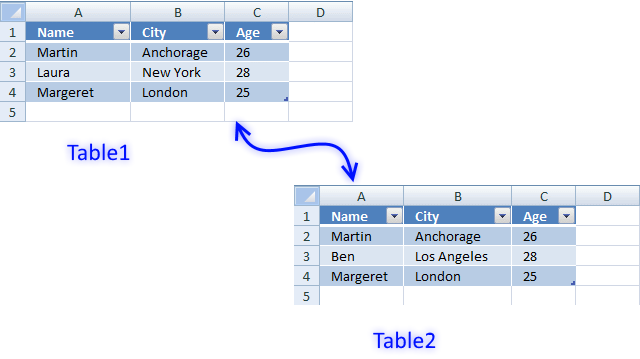This article demonstrates how to filter records occurring in only one out of two Excel defined tables. It also shows you an array formula that extracts records not shared by both data sets.

Let me demonstrate how to extract non-common records from two tables.

The formulas in this post contain the COUNTIFS function and were introduced in Excel 2007.

The first example shows you how to hide common rows in a table.

The second example describes how to compare two tables and extract not common rows/records using an array formula.

### Example 1 - Excel defined tables

Sheet 1 and sheet 2 contains random data. As you can see, row 1 and 3 are common records between the two tables.

Count records using COUNTIFS function

1. Select cell D2 in sheet1.
2. Type
=COUNTIFS(Sheet2!\$A\$2:\$A\$4, A2, Sheet2!\$B\$2:\$B\$4, B2, Sheet2!\$C\$2:\$C\$4, C2)

in cell D2.

3. Press Enter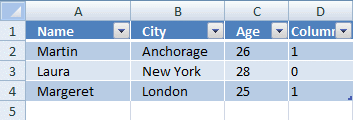The formula is instantly copied to all table cells in column D. The countifs function counts common records from two tables. Row 2 in sheet1 is found once in sheet 2 and so on.

Hide common rows

1. Press with mouse on black arrow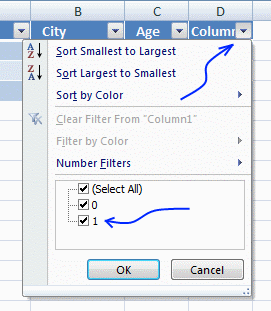2. Deselect 1.
3. Press with left mouse button on OK.

Common records are removed.Repeat steps in Count records using COUNTIFS function and Hide common rows with sheet2.

### Example 2 - Extract records using an array formula

This example describes how to compare two tables and extract not common rows/records using an array formula.

Sheet1Sheet2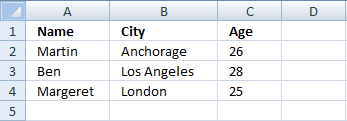Compare sheet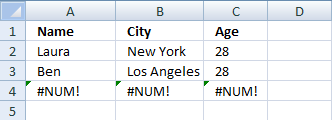Array formula in cell A2:

=IFERROR(INDEX(Sheet1!\$A\$2:\$C\$4, SMALL(IF(COUNTIFS(Sheet1!\$A\$2:\$A\$4, Sheet2!\$A\$2:\$A\$4, Sheet1!\$B\$2:\$B\$4, Sheet2!\$B\$2:\$B\$4, Sheet1!\$C\$2:\$C\$4, Sheet2!\$C\$2:\$C\$4)=0, MATCH(ROW(\$A\$2:\$A\$4), ROW(\$A\$2:\$A\$4)), ""), ROW(A1)), COLUMN(A1)), INDEX(Sheet2!\$A\$2:\$C\$4, SMALL(IF(COUNTIFS(Sheet2!\$A\$2:\$A\$4, Sheet1!\$A\$2:\$A\$4, Sheet2!\$B\$2:\$B\$4, Sheet1!\$B\$2:\$B\$4, Sheet2!\$C\$2:\$C\$4, Sheet1!\$C\$2:\$C\$4)=0, MATCH(ROW(\$A\$2:\$A\$4), ROW(\$A\$2:\$A\$4)), ""), ROW(A1)-SUM(--(COUNTIFS(Sheet1!\$A\$2:\$A\$4, Sheet2!\$A\$2:\$A\$4, Sheet1!\$B\$2:\$B\$4, Sheet2!\$B\$2:\$B\$4, Sheet1!\$C\$2:\$C\$4, Sheet2!\$C\$2:\$C\$4)=0))), COLUMN(A1)))

This array formula may look complicated but it is not. It contains two different formulas. If the first formula returns an error, the second formula is calculated. The first formula extracts not common rows from the first table. The second formula extracts not common rows from the second table.

You can add a second IFERROR() function to the formula to remove #num errors.

### How to create an array formula

1. Copy (Ctrl + c) and paste (Ctrl + v) array formula into formula bar.2. Press and hold Ctrl + Shift.
3. Press Enter once.
4. Release all keys.

### Explaining formula

This formula is the first formula. It extracts not common rows from the first table. The second formula is exactly the same but cell references are pointing to the second table.

INDEX(Sheet1!\$A\$2:\$C\$4, SMALL(IF(COUNTIFS(Sheet1!\$A\$2:\$A\$4, Sheet2!\$A\$2:\$A\$4, Sheet1!\$B\$2:\$B\$4, Sheet2!\$B\$2:\$B\$4, Sheet1!\$C\$2:\$C\$4, Sheet2!\$C\$2:\$C\$4)=0, MATCH(ROW(\$A\$2:\$A\$4), ROW(\$A\$2:\$A\$4)), ""), ROW(A1)), COLUMN(A1))

Step 1 - Identify common records

COUNTIFS(criteria_range1,criteria1, criteria_range2, criteria2...)
Counts the number of cells specified by a given set of conditions or criteria

COUNTIFS(Sheet1!\$A\$2:\$A\$4, Sheet2!\$A\$2:\$A\$4, Sheet1!\$B\$2:\$B\$4, Sheet2!\$B\$2:\$B\$4, Sheet1!\$C\$2:\$C\$4, Sheet2!\$C\$2:\$C\$4)=0

becomes

COUNTIFS({"Martin";"Laura";"Margeret"}, {"Martin";"Ben";"Margeret"}, {"Anchorage";"New York";"London"}, {"Anchorage";"Los Angeles";"London"}, {26;28;25}, {26;28;25})=0

becomes

{1;0;1}=0 and returns {FALSE; TRUE; FALSE}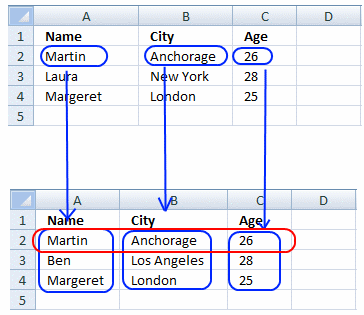Step 2 - Convert array into row numbers

IF(COUNTIFS(Sheet1!\$A\$2:\$A\$4, Sheet2!\$A\$2:\$A\$4, Sheet1!\$B\$2:\$B\$4, Sheet2!\$B\$2:\$B\$4, Sheet1!\$C\$2:\$C\$4, Sheet2!\$C\$2:\$C\$4)=0, MATCH(ROW(\$A\$2:\$A\$4), ROW(\$A\$2:\$A\$4)), "")

becomes

IF({FALSE; TRUE; FALSE}, MATCH(ROW(\$A\$2:\$A\$4), ROW(\$A\$2:\$A\$4)), "")

becomes

IF({FALSE; TRUE; FALSE}, MATCH({2;3;4}, {2;3;4}), "")

becomes

IF({FALSE; TRUE; FALSE}, {1;2;3}), "")

and returns

{"";2;""}

Step 3 - Return the k-th smallest number

SMALL(array,k) returns the k-th smallest number in this data set.

SMALL(IF(COUNTIFS(Sheet1!\$A\$2:\$A\$4, Sheet2!\$A\$2:\$A\$4, Sheet1!\$B\$2:\$B\$4, Sheet2!\$B\$2:\$B\$4, Sheet1!\$C\$2:\$C\$4, Sheet2!\$C\$2:\$C\$4)=0, MATCH(ROW(\$A\$2:\$A\$4), ROW(\$A\$2:\$A\$4)), ""), ROW(A1))

becomes

SMALL({"";2;""}, ROW(A1))

becomes

SMALL({"";2;""}, 1)

and returns 2.

Step 4 - Return a value of the cell at the intersection of a particular row and column

INDEX(array,row_num,[column_num])
Returns a value or reference of the cell at the intersection of a particular row and column, in a given range

INDEX(Sheet1!\$A\$2:\$C\$4, SMALL(IF(COUNTIFS(Sheet1!\$A\$2:\$A\$4, Sheet2!\$A\$2:\$A\$4, Sheet1!\$B\$2:\$B\$4, Sheet2!\$B\$2:\$B\$4, Sheet1!\$C\$2:\$C\$4, Sheet2!\$C\$2:\$C\$4)=0, MATCH(ROW(\$A\$2:\$A\$4), ROW(\$A\$2:\$A\$4)), ""), ROW(A1)), COLUMN(A1))

becomes

INDEX(Sheet1!\$A\$2:\$C\$4, 2, COLUMN(A1))

becomes

INDEX(Sheet1!\$A\$2:\$C\$4, 2, 1)

becomes

INDEX({"Martin", "Anchorage",26;"Laura", "New York", 28;"Margeret", "London", 25}, 2, 1)

and returns "Laura" in cell A2.

### Get the Excel fileremove-common-records.xlsx

## Compare two tables using a condition

There are two tables on this sheet. The array formula extracts common records in the two tables using a condition. There can be numerous occasions where this can be useful, for example comparing values on the same year or maybe year and month. The picture below shows common names in region Asia.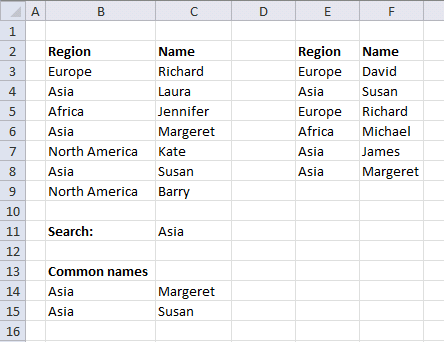Array formula in cell B14:

=INDEX(\$B\$3:\$C\$9, SMALL(IF(COUNTIFS(\$E\$3:\$E\$8, \$B\$3:\$B\$9, \$F\$3:\$F\$8, \$C\$3:\$C\$9)*(\$B\$3:\$B\$9=\$C\$11), MATCH(ROW(\$B\$3:\$B\$9), ROW(\$B\$3:\$B\$9)), ""), ROW(A1)), COLUMN(A1))

How to enter an array formula

1. Select cell B14
2. Paste formula to formula bar3. Press and hold CTRL + SHIFT
4. Press ENTER

There are now curly brackets surrounding the formula in the formula bar.

{=array_formula}

### Defined tables

This example demonstrates how to filter not common values in region Asia using defined tables. I converted the two tables to excel defined tables.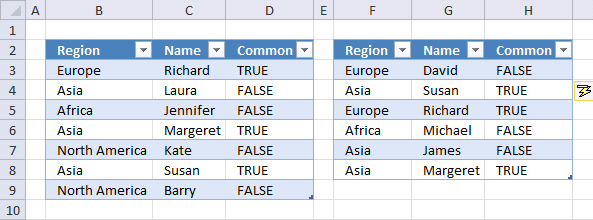Formula in cell D3:

=COUNTIFS(Table2[[Region ]],B3,Table2[Name],C3)>0

Formula in cell H3:

=COUNTIFS(Table1[Region],F3,Table1[Name],G3)>0

Filter values

1. Press with left mouse button on black arrow near header "Common"
2. Deselect True
3. Press with left mouse button on Ok
4. Press with left mouse button on
5. Press with left mouse button on black arrow near header "Region"
6. Deselect all values except "Asia"
7. Press with left mouse button on OK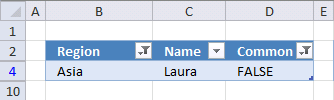### Get the Excel fileCompare-two-tables-using-a-condition.xlsx